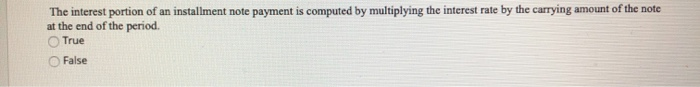# The interest portion of an installment note payment is computed byThe interest portion of an installment note payment is computed by multiplying the interest rate by the carrying amount of the note at the end of the period. True False

Solution :
True, The interest portionof an installment
note payment is computed by multiplying the interest rate
by the carrying amount of the note at the end of the
period. This can be justified by the following example
:
e.g : Business borrows 40,000 at the start of an accounting
period (January 1) by signing a 5% installment note that is to be
repaid in 4 annual end of year payments of 11,280. The first
payment is due at the end of the accounting period (December
31).

Calculation of Interest each year
Year 1=40000*5%=2000
Year 2=30720*5%1536
Year 3=20975*5%=1049
Year 4=10743*5%=537

 Particulars Year 1 Year 2 Year 3 Year 4 Opening 40000 30720 20975 10743 [email protected]% 2000 1536 1049 537 Fixed Installment 11280 11280 11280 11280 Closing 30720 20975 10743 0 Principal 9280 9744 10232 10743